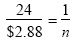Multiple Choice
Identify the choice that best completes the statement or answers the question.

1.

Number Sense and Numeration – Decimals – RIT 221 – 230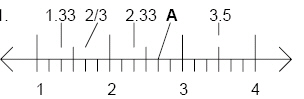Which value correctly represents point A?
 a. 2 1/6 d. 2 2/3 b. 2 1/3 e. 2 5/6 c. 2.5

2.

Number Sense and Numeration – Exponents – RIT 221 – 230

Fill in the boxes from left to right with the correct exponent.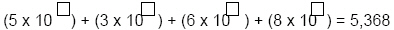a. 4, 3, 2, 1 d. 0, 1, 2, 3 b. 3, 2, 1, 0 e. 5, 3, 6, 8 c. 1, 2, 3, 4

3.

Number Sense and Numeration – Fractions – RIT 221 – 230

Write in simplest form.
In a bag of 70 jellybeans, 45 are green. What fraction of the bag of jellybeans
is green?
 a. 14/9 d. 25/70 b. 70/45 e. 45/70 c. 9/14

4.

What is the ratio of squares to triangles?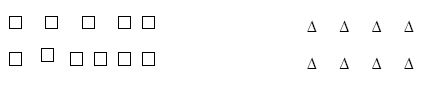a. 8 : 11 d. 4 to 3 b. 11 : 8 e. even to odd c. 1 to 1

5.

Choose the pair of fractions that is equivalent to each other.
 a. 3/2 and 1/2 d. 2/3 and 1/3 b. 6/4 and 7/4 e. 9/6 and 3/2 c. 8/12 and 4/12

6.

Fill in the box that would make the two ratios equivalent.

4   =    1620
 a. 16 d. 8 b. 5 e. 80 c. 10

7.

Use cross-products to determine which of the following is true:
 a. 2/3 = 5/7 d. 4/5 = 6/7 b. 1/8 = 8/1 e. 5/24 = 7/18 c. 8/12 = 4/6

8.

Number Sense and Numeration – Integers – RIT 221 – 230

Which of the following is an integer?
 a. –3/4 d. –2 2/3 b. –8 e. –5.3 c. –0.56

9.

Number Sense and Numeration – Ordering, Equalities and Inequalities –
RIT 221 – 230

Which statement is in the correct order from least to greatest?
 a. –9, -11, -14, -17, -24 d. –6, -7, -12, 0, 1 b. 3, -4, 7, -14, -15 e. –15, 13, 11, -7, -9 c. –27, -14, -1, 0, 13

10.

Number Sense and Numeration – Percents – RIT 221 – 230

Which of the following is a true statement?
 a. 56/100 = 56% d. 6/100 = 1/6% b. 6/100 = 60% e. 1/100 = 100% c. 75% = 75/1000

11.

Number Sense and Numeration – Place Value – RIT 221 – 230

Which decimal represents six and twenty-five thousand, seven hundred
eighty-four hundred-thousandths?
 a. 6.25701 d. 6.784 b. 6.25001 e. 6.25784 c. 6.2784

12.

Number Sense and Numeration – Square Roots – RIT 221 – 230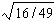a.d.b.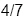e.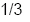c.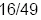13.

Billy starts soccer at 2:45 P.M. He practices for 35 minutes. What time
does practice end?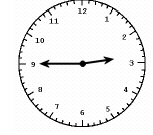a. 3:15 d. 3:20 P.M. b. 4:00 P.M. e. 3:30 A.M. c. 3:15 P.M.

14.

What is the probability that the spinner will land on yellow?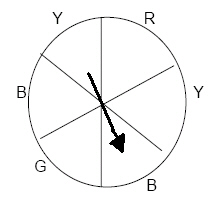a. 2 out of 4 d. 1 out of 4 b. 1 out of 6 e. 4 out of 6 c. 2 out of 6

15.

The Wongs are going to fence their yard. How many feet of fence do they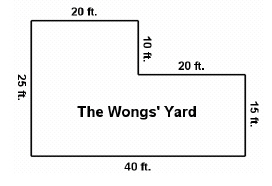a. 100 feet d. 120 feet b. 110 feet e. 130 feet c. 65 feet

16.

9, 767, 422, 135
What is the value of the digit in the one billions place?
 a. 9 d. 9,000 b. 9,000,000,000 e. 900 c. 9,000,000

17.

Diana received scores of 100, 63, 90, 85, and 92 on her math tests. What
is her mean (average) score?
 a. 81 d. 415 b. 82 e. 85 c. 83

18.

There are 60 marbles in a jar. If 8 children get the same number of
marbles, how many will be left over?
 a. 52 d. 4 b. 8 e. 0 c. 11

19.

A jacket costs \$25. It is on sale for a 20% discount. How much is the sale
price?
 a. \$5.00 d. \$20.00 b. \$30.00 e. \$45.00 c. \$50.00

20.

Betty’s Bakery sells chocolate chip, oatmeal, and peanut butter cookies.
They come in two sizes, regular and jumbo. What are the possible
 a. regular chocolate chip, regular peanut butter, regular oatmeal b. regular chocolate chip, regular peanut butter, jumbo chocolate chip,jumbo peanut butter c. jumbo chocolate chip, jumbo peanut butter, jumbo oatmeal d. regular chocolate chip, regular peanut butter, regular oatmeal,jumbo chocolate chip, jumbo peanut butter, jumbo oatmeal e. oatmeal, chocolate chip, peanut butter

21.

If you have two tables that seat 6 people each, how many people can you
seat if you push the two tables together?a. 12 d. 11 b. 8 e. 10 c. 6

22.

.–11 + 16 = 16 – 11
Which property does this demonstrate?
 a. Commutative d. Distributive b. Associative e. Zero c. Identity

23.

Calculate  122
 a. 14 d. 144 b. 10 e. 5 c. 24

24.

Use proportions to solve the following problem.
If a package of 24 pencils costs \$2.88, how much will 1 pencil cost?

 a.d.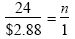b.e.c.